Newton's Law of Viscosity and Equation | Mecholic -->

# Newton's Law of Viscosity and Equation## Newton’s law of viscosity

Newton’s law of viscosity states that the stress on fluid layers is directly proportional to the rate of shear strain.
Mathematically,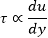## Dynamic Viscosity (μ)

Viscosity is defined as the measure of fluid resistance to the flow of one layer of fluid over adjacent layer. Fig shows two fluid layers at distance y and y+dy from the surface. They move with different velocities u and u+du as shown in fig. The top layer causes a shear stress on lower while lower layer causes shear stress on the top layer. The shear stress τ is proportional to the rate of change of velocity with respect y.
Mathematically,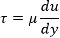Here constant of proportionality μ is known as the coefficient of dynamic viscosity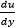known as the velocity gradient.

From the above equation,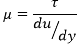Unit of dynamic viscosity
In SI: Newton-Sec/m2 = NS/m2
In CGS: dyne-Sec/cm2
1 dyne-Sec/cm2 called one poise.
One poise = 0.1 NS/m2

## Kinematic viscosity (ν)

It is defined as the ratio between dynamic viscosity and density of the fluid.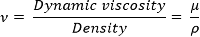Units of Kinematic viscosity
In SI system: m2/s
In CGS: cm2/s,
One cm2/s known as Stoke
One stoke = 10-4 m2/s

Remember
• For a Newtonian fluid, the coefficient of viscosity remains constant.
• The viscosity of a liquid decreases with increase in temperature.
• The viscosity of gases increases with increase in temperature.
• Material in the increasing order of their viscosity: gasoline < water < crude oil < castor oil
-What is meant by viscosity
- What Does Surface Tension Mean
- Properties of Fluids